# Two cars

Two cars started against each other at the same time to journey long 346 km. The first car went 53 km/h and the second 53 km/h.

What distance will be between these cars 20 minutes before meet?

x =  35.33 km

### Step-by-step explanation: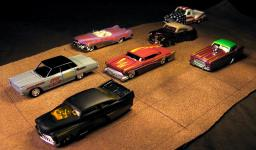Did you find an error or inaccuracy? Feel free to write us. Thank you!Math studentMatematik
Thank you for your feedback, but we don't know what in the problem solution is wrong ( spelling - text of the problem, calculation, numeric  error, ...) Please describe more...Tips to related online calculators
Do you have a linear equation or system of equations and looking for its solution? Or do you have a quadratic equation?
Do you want to convert length units?
Do you want to convert velocity (speed) units?
Do you want to convert time units like minutes to seconds?

## Related math problems and questions:

• Against each other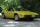From two points A, B distant 23 km at the same time started two cars against each other at speeds 41 km/h and 65 km/h. How long does cars meet and what distance passes each of them?
• Cars 2Opava is 360 km distance from Prešov. At 8:00 hour, two cars started against each other from this cities at average speeds 88 km/h and 121 km/h. What hour will it meet and what distance it's travel if the car started from Prešov is faster?
• Cars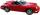Cars started at 7:00 AM. From point A car run at 40 km per hour, from B car run 70 km per hour. Between A and B is a 250 km long road. When and where will meet (measured from point A)?
• TruckIn 7 hours started from town Krnov truck at speed 40 km/h. Passenger car started against it in 8 hours 30 minutes from the city of Jihlava at speed 70 km/h. Distance between this two cities is 225 km. At what time and at what distance from Krnov this two
• Two trains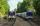Two trains go at each other. First at speed 40 km/h and the second at speed 60 km/h. At what distance will be 30 minutes before they will meet?
• Motion 2At 10 o'clock started from place A car speed at 90 km/h. 20 minutes after started from the place B at speed 60 km/h another car. Distance places A and B is 120 kilometers. What time and where they meet?
• Two friendsTwo friends on a bicycle rode against each other from two places 49 km apart. The first set off at 8.00 at a speed of 20km/h, the second 12 minutes later at a speed of 25km/h. What time do they meet? How many km will each of them travel since then?
• Cars motion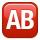From point A car start at the speed of 90 km/h. Against it departs from the point B at the same time another car at 60 km/h. For how long and where they met if the distance between A and B is 180 km?
• Motion problemFrom Levíc to Košíc go car at speed 81 km/h. From Košíc to Levíc go another car at speed 69 km/h. How many minutes before the meeting will be cars 27 km away?
• Two citiesCities A and B are 200 km away. At 7 o'clock from city A, the car started at an average speed of 80 km/h, and from B at 45 min later, the motorcycle is started at an average speed of 120 km/h. How long will they meet, and at what distance from point A wil
• Car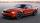The car goes from point A to point B at speed 86 km/h and back 53 km/h. If it goes there and back at speed 67 km/h trip would take 10 minutes shorter. What is the distance between points A and B?
• MotionFrom two different locations distant 232 km started against car and bus. The car started at 5:20 with average speed 64 km/h. Bus started at 7:30 with average speed 80 km/h. When they meet? How many kilometers went the bus?
• TroopsThe route is long 147 km and the first-day first regiment went at an average speed of 12 km/h and journey back 21 km/h. The second day went second regiment the same route at an average speed of 22 km/h there and back. Which regiment will take route longer
• Pedestrian and the cyclist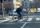Pedestrian and cyclist went against each from the two cities that are distant 40 km. Pedestrian started 30 minutes before the cyclist and walk an average speed of 5 km/h. What speed went cyclist when met after 120 minutes from the start of the pedestrian?
• Two trains meetFrom A started at 7:15 express train at speed 85 km/h to B. From B started passenger train at 8:30 in the direction to A and at speed 55 km/h. The distance A and B are 386 1/4 km. At what time and at what distance from B the two trains meet?
• Two cars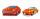From the town A to town B started two cars. The first at 7:00 at average speed 60 km per hour, the second at 10:00 at average speed 100 km per hour. The first car will not stay in B, and on the way back meet the second car at half way from A to B. At what
• CarThe driver of a car is to get to 608 km distant city. From atlas found that 162 km will have to pass through the cities at an average speed 48 km/h. The remainder of the journey passes outside the cities at average speed 116 km/h. Calculate how many hours